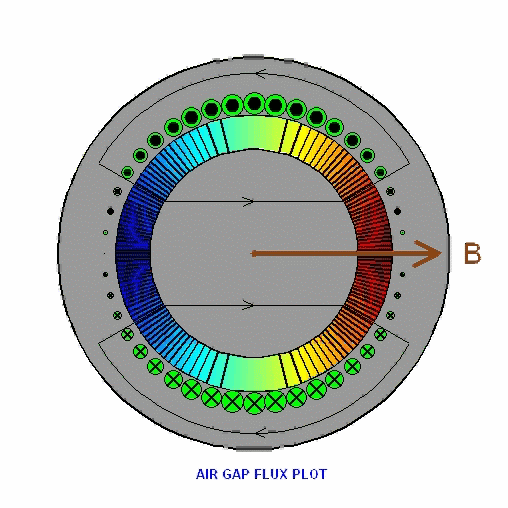﻿ Air-gap flux plot

RETURN

FLUX PLOT

A rotating sinusoidally distributed winding is excited with a constant current. The flux plot in the air gap also rotates. Where the lines are crowded, the corresponding magnetic field density B is high, and where the concentration of lines is reduced so is B. Thus the air gap B-field is sinusoidally distributed in space and is also rotating. It can be represented by a rotating space vector of constant magnitude pointing to where the field is maximum positive.